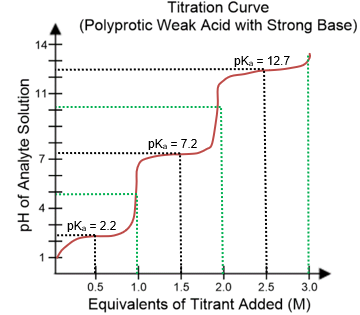Clutch Prep is now a part of Pearson

# Titration

See all sections
Sections
Properties of Water
Osmosis
Hydrophobic Effect
Acids and Bases
Autoionization of Water
pH
Acid Dissociation Constant
Henderson Hasselbalch Equation
Determining Predominate Species
Titration
Buffer Solution

Concept #1: Understanding Titrations

Practice: Which of the following titration curves expresses the titration of a weak acid with a strong base?

Concept #2: Titration of Weak Acids

Practice: You have an analyte solution of 50 mL of 0.2 M acetic acid (pKa = 4.8). What volume of 0.05 M NaOH titrant needs to be added to get the final pH = pKa?

Concept #3: Titration of Polyprotic Weak Acids

Practice: Use the titration curve below.What is the predominate species in the solution of phosphoric acid at pH = 5?

Practice: Titration confirms an acetic acid solution to be 0.1 M. Calculate the pH. (acetic acid K a = 1.76 x 10-5 M).